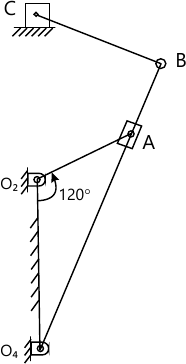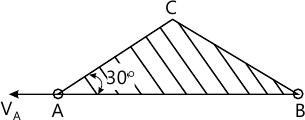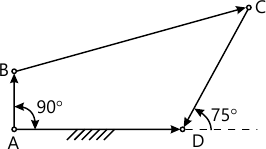MORE IN Kinematics of Machinery
VTU Mechanical Engineering (Semester 4)
Kinematics of Machinery
June 2013
Total marks: --
Total time: --
INSTRUCTIONS
(1) Assume appropriate data and state your reasons
(2) Marks are given to the right of every question
(3) Draw neat diagrams wherever necessary

1 (a) Define: i) Machine and ii) Mechanism. State an example for each.
6 M
1 (b) Five binary links of lengths 5cm, 8cm, 15cm, 19cm and 28cm are available for constructing a crank-rocker mechanism. Select four links required for the construction of this mechanism. Sketch (need not be to scale) the mechanism and clearly show the fixed link, crank and rocker.
6 M
1 (c) Sketch a 'Double slider crank chain'. A mechanism is obtained from this by fixing a binary link having two turning pairs. State an application for this mechanism and sketch the same.
8 M

2 (a) The length of the fixed link of a crank and slotted-lever mechanism (quick return motion) is 250 mm and that of the crank is 100 mm. Determine i) angle between extreme positions of slotted lever and ii) Ratio of the time of cutting stroke to that of return stroke.
6 M
2 (b) Draw a neat proportionate 'Peaucellier Mechanism'. Indicate the geometric relations among the link and show the point tracing the straight line. Prove that the point traces a straight line perpendicular to the fixed link.
14 M

3 In the slotted-lever quick-return mechanism shown in Fig Q3, the crank O2A rotates at constant speed of 30 RPM (CCW). For the position shown determine the velocity and acceleration of the point C. Given O2A=12cm, O2O4=30 cm, O4B=60 cm and BC=15cm. The line of movement of C is 30cm above the point O2.20 M

4 (a) What is instantaneous centre of rotation? Stake Kennedy's theorem.
6 M
4 (b) A binary link of a mechanism having two turning pairs at A and B is in the shape of an isosceles triangles as shown in Fig Q4(b) (complete mechanism is not shown). Velocity of A is 20 cm/s along AB in the direction shown in the Fig Q4(b). Determine the instantaneous centre of the link if the path of B at the instant is along BC. Also determine the magnitude and direction of, i) angular velocity of the link ii) Velocity of C.6 M
4 (c) Explain the procedure to construct Klein's construction to determine the velocity and acceleration of a slider Crank mechanism in which crank is rotating uniformly.
8 M

5 In the 4-bar mechanism shown in Fig Q5, link AB rotates uniformly at 2 radians per second in clockwise sense. Using complex algebra write loop closure equation for this. Determine magnitude and directions of angular velocity and angular acceleration of links BC and CD using vector algebra. Also state whether the magnitudes of angular velocity of these links tend to increase or decrease at the instant.20 M

6 (a) State and prove law of gearing.
8 M
6 (b) The number of teeth on each of the two equal spur gear in mesh is 40. The teeth have 20° involute profile and the module is 6 mm. If the length of are of contact is 1.75 times the circular pitch, find the addendum.
8 M
6 (c) Compare involute and cycloidal tooth profile of a gear with respect to, i) Pressure angle ii) Interference.
4 M

7 An epicyclic gear train has a fixed annular wheel 'C' concentric with sun wheel 'A'. A planet wheel 'B' gears with 'A' and 'C' and can rotate freely on a pin carried by an arm 'D' which rotates about an axis co-axial with that of 'A' and 'C'. If T1 and T2 are the numbers of teeth on 'A' and 'C' respectively show that the ratio of speed of 'D' to 'A' is $\dfrac {T_1} {T_1+T_2}$ If the least number of teeth on any wheel is 18 and T1+T2=120, find the greatest and least speeds of D when wheel 'A' rotates at 500 RPM.
20 M

8 (a) Draw neat sketch for each of the following:
i) Plate or disc cam with a translation follower
ii) Wedge cam with translating follower
iii) Cylindrical cam with translating follower.
6 M
8 (b) Draw the profile of a cam operating a knife-edge follower having a lift of 30 mm. The cam raises the follower with SHM for 150° of the rotation followed by a period of dwell for 60°. The follower descends for the next 100° rotation of the cam with uniform velocity, again followed by a dwell period. The cam rotates in an anticlockwise sense at a uniform velocity of 120 RPM and has a least radius of 25mm. What will be the maximum velocity and acceleration of the follower during the lift.
14 M

More question papers from Kinematics of Machinery